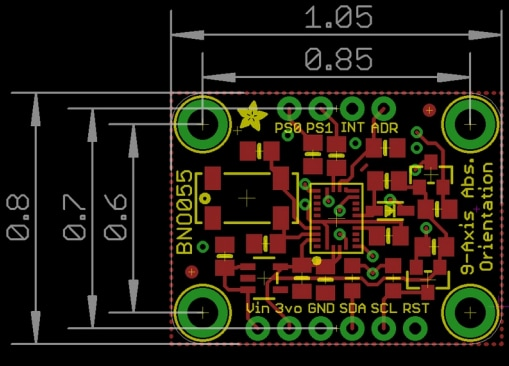# Adafruit BNO055 IMU Fusion Breakout - Stemma QT

Adafruit BNO055 IMU Fusion Breakout - Stemma QT is a 9-DoF absolute orientation Inertial Measurement Unit (IMU) fusion breakout sensor. This IMU outputs the following sensor data like absolute orientation, angular velocity vector, acceleration vector, magnetic field strength vector, linear acceleration vector, gravity vector, and temperature. The BNO055 IMU breakout processes the sensor data, abstracts the sensor fusion and real-time requirements away, and outputs data in quaternions, Euler angles, and vectors. This IMU breakout also features STEMMA QT connectors for the I2C bus and is 3V and 5V logic safe.

## Features

• Absolute Orientation (Euler Vector, 100Hz):
• Three-axis orientation data based on a 360° sphere
• Absolute Orientation (Quatenrion, 100Hz):
• Four-point quaternion output for more accurate data manipulation
• Angular Velocity Vector (100Hz):
• Three-axis of 'rotation speed' in rad/s
• Acceleration Vector (100Hz):
• Three-axis of acceleration (gravity + linear motion) in m/s^2
• Magnetic Field Strength Vector (20Hz):
• Three-axis of magnetic field sensing in micro Tesla (uT)
• Linear Acceleration Vector (100Hz):
• Three-axis of linear acceleration data (acceleration minus gravity) in m/s^2
• Gravity Vector (100Hz):
• Three-axis of gravitational acceleration (minus any movement) in m/s^2
• Temperature (1Hz):
• Ambient temperature in degrees celsius

## Board Dimensionsเผยแพร่แล้ว: 2563-08-14 | อัปเดตแล้ว: 2565-09-13